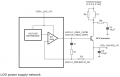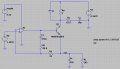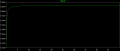# Need help understanding this LDO topology

#### alfhg

Joined May 10, 2019
3
I'm trying to understand how a LDO topology works. It's in NXP IC's datasheet:
It has the control circuitry inside the IC with external transistor.Parameters:
VDD output = 1.8V
Collector supply = 2.367V
NPN: Nexperia PBSS4620PA
L1: ferrite bead 26 Ohm @ 100MHz
C1 = 470nF
Rp = 1 Ohm
Rs = 0.15 Ohm
R1 = 49.9 Ohm
R2 = 18 Ohm
Voltage reference is supposedly a bandgap reference at 1.2V.

This topology isn't like the typical LDO, where the error amplifier feedback is taken from a voltage divider at Vout. In this circuit R1 and R2 don't have a ground path, but connect back to BJT base with AC coupling. How is this supposed to work?

I ran an LTSpice simulation to find the DC load curve. The result doesn't even make sense.
LTspice schematic:
(U1 is UniversalOpAmp generic model without input and output limits)Vout vs RL waveform:This doesn't make sense, because it's an LDO, Vout is expected to be always 1.8V with varying RL.

How should this work?

#### ericgibbs

Joined Jan 29, 2010
17,180
hi alf,
Welcome to AAC.
E

#### crutschow

Joined Mar 14, 2008
31,580
This doesn't make sense, because it's an LDO, Vout is expected to be always 1.8V with varying RL.
It makes sense.
In order for the voltage between the two op amp differential inputs be essentially 0V at balance with a 1.205V reference, the output will also be 1.205V due to the DC feedback loop through R2.

Makes no sense that you expect it to be 1.8V(?).

#### alfhg

Joined May 10, 2019
3
hi alf,
Welcome to AAC.
E
I can't find where to edit the original post. So I'm attaching LTSpice schematic and BJT library files in a reply.

#### Attachments

• 2.2 KB Views: 1
• 1.5 KB Views: 0
• 530 bytes Views: 0

#### alfhg

Joined May 10, 2019
3
It makes sense.
In order for the voltage between the two op amp differential inputs be essentially 0V at balance with a 1.205V reference, the output will also be 1.205V due to the DC feedback loop through R2.

Makes no sense that you expect it to be 1.8V(?).
Yes, I get the point, but according to NXP, it's using the same internal voltage reference (assumed 1.2V) to generate both 1.8V and 1.1V, with Vout determined by R1 and R2.
The suggested values for different Vout:
For Vout = 1.8V: R1 = 50, R2 = 18
For Vout = 1.1V: R1 = 110, R2 = 6.8
The datasheet is a new part provided under NDA, I'm not supposed to post it in public.

#### crutschow

Joined Mar 14, 2008
31,580
The suggested values for different Vout:
For Vout = 1.8V: R1 = 50, R2 = 18
For Vout = 1.1V: R1 = 110, R2 = 6.8
Obviously that won't work as shown since there is no DC path for R1.
Adding a resistor to ground from the left side of R2 would increase the output voltage, but I see no obvious way to get the output voltage below the reference voltage, unless an attenuator is added to the reference voltage.

Last edited:

#### DickCappels

Joined Aug 21, 2008
9,632
Can you post the NXP data sheet or the part number associated with it. Either it is wrong or being misunderstood.